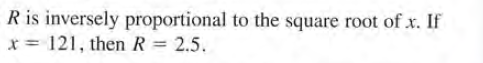# R is inversely proportional to the square root of x. If x = 121, then R = 2.5.

Question

Express the statement as an equation. Use the given information to find the constant of proportionality.help_outlineImage TranscriptioncloseR is inversely proportional to the square root of x. If x = 121, then R = 2.5. fullscreen

### Want to see this answer and more?

Experts are waiting 24/7 to provide step-by-step solutions in as fast as 30 minutes!*

*Response times may vary by subject and question complexity. Median response time is 34 minutes for paid subscribers and may be longer for promotional offers.
Tagged in
Math
Algebra

### Applications of Mathematics